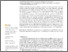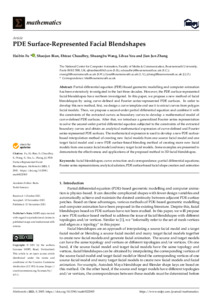# Pde surface-represented facial blendshapes.

Fu, H., Bian, S., Chaudhry, E., Wang, S., You, L. and Zhang, J. J., 2021. Pde surface-represented facial blendshapes. Mathematics, 9 (22), 2905.

Full text available as:Preview
PDF (OPEN ACCESS ARTICLE)
mathematics-09-02905-v2.pdf - Published Version

27MB

## Abstract

Partial differential equation (PDE)-based geometric modelling and computer animation has been extensively investigated in the last three decades. However, the PDE surface-represented facial blendshapes have not been investigated. In this paper, we propose a new method of facial blendshapes by using curve-defined and Fourier series-represented PDE surfaces. In order to develop this new method, first, we design a curve template and use it to extract curves from polygon facial models. Then, we propose a second-order partial differential equation and combine it with the constraints of the extracted curves as boundary curves to develop a mathematical model of curve-defined PDE surfaces. After that, we introduce a generalized Fourier series representation to solve the second-order partial differential equation subjected to the constraints of the extracted boundary curves and obtain an analytical mathematical expression of curve-defined and Fourier series-represented PDE surfaces. The mathematical expression is used to develop a new PDE surface-based interpolation method of creating new facial models from one source facial model and one target facial model and a new PDE surface-based blending method of creating more new facial models from one source facial model and many target facial models. Some examples are presented to demonstrate the effectiveness and applications of the proposed method in 3D facial blendshapes.

Item Type: Article 2227-7390 Faculty of Media & Communication 36313 Symplectic RT2 29 Nov 2021 15:59 14 Mar 2022 14:30